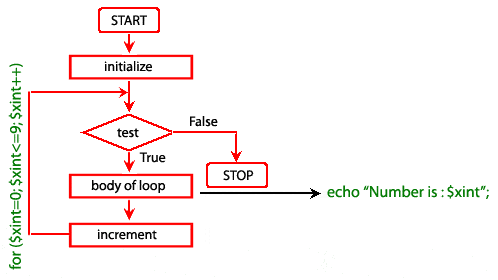# Education is not limited to just classrooms. It can be gained anytime, anywhere... - Ravi Ranjan (M.Tech-NIT)

## PHP for Loop

Description

The PHP for loop allows the user to put all the loop-related statements (i.e. INITIALIZER; CONDITION; INCREMENTOR or DECREMENTOR) in one place. The structure is similar to C language.

Syntax

for ( initialize counter ; test counter ; increment counter)

{

execute the statement;

}

• initialize counter : Initialize the loop counter value.

• test counter : Verify the loop counter whether the condition is true.

• increment counter : Increasing the loop counter value.

• execute the statement : Execute php statements.

Alternatively, you can write for loop statement using the following syntax also.

for (expr1; expr2; expr3):

statement

...

endfor;

Pictorial representation of for loopExample :

The following example displays the numbers between 0 to 5.

view plaincopy to clipboardprint?

1. for (\$xint=0; \$xint<=5; \$xint++)

2. {

3. echo "Number is : \$xint
";

4. }

5. ?>

Output :

Number is : 0
Number is : 1
Number is : 2
Number is : 3
Number is : 4
Number is : 5

You can execute for in the following ways also.

view plaincopy to clipboardprint?

1. for (\$xint = 1; ; \$xint++) {

2. if (\$i >= 5) {

3. break;

4. }

5. echo \$xint;

6. }

7. ?>

view plaincopy to clipboardprint?

1. \$xint = 1;

2. for (; ; )

3. {

4. if (\$xint >= 5)

5. {

6. break;

7. }

8. echo \$xint;

9. \$xint++;

10. }

11. ?>

Example : Counting number of times a word present in a sentence.

view plaincopy to clipboardprint?

1. \$text="The quick brown Fox jumps over the lazy Dog";

2. \$words = explode(" ", \$text); // explode function looks for " "  and creates an array, where each word is an element of the array

3. \$now = count(\$words);

4. \$j = 0;

5. for(\$i=0; \$i<\$now; \$i++)

6. {

7. if (\$words[\$i] == "the" or \$words[\$i] == "The")

8. {

9. \$j =  \$j+1;

10. }

11. }

12. echo \$j;

13. ?>

Output :

2